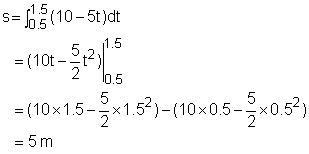Ch 6. Integrals Multimedia Engineering Math IndefiniteIntegral Area DefiniteIntegral FundamentalTheorem SubstitutionRule
 Chapter 1. Limits 2. Derivatives I 3. Derivatives II 4. Mean Value 5. Curve Sketching 6. Integrals 7. Inverse Functions 8. Integration Tech. 9. Integrate App. 10. Parametric Eqs. 11. Polar Coord. 12. Series Appendix Basic Math Units Search eBooks Dynamics Fluids Math Mechanics Statics Thermodynamics Author(s): Hengzhong Wen Chean Chin Ngo Meirong Huang Kurt Gramoll ©Kurt GramollMATHEMATICS - CASE STUDY SOLUTION The car has an initial speed of 10m/s when the traffic light turns red and starts to decelerate at -5 m/s2. How far does the car travel before it stops? Let the velocity, distance and time to be v, s and t respectively. The velocity at 0 and t second is represented by v0 and vt respectively. In order to find the traveling distance, the time spent on stopping the car should to be calculated first. The velocity function is      vt = v0 + at in which v0 = 10, vt = 0 and a = -5. Substituting v0, v0 and a into the above equation gives      t = 2.0 The stopping distance is the integral of velocity with respect to time, that is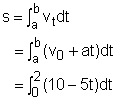In the above equation, the lower limit is the time that the car starts to brake, which is 0 seconds. The upper limit is the time that the car stops, which is 2 seconds.The Fundamental Theorem of Calculus gives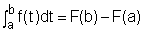The distance the car travels can be calculated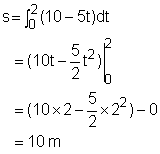Discussion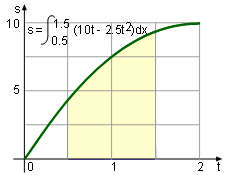Braking Distance in [0.5, 1.5] The braking distance for different intervals can be calculated by changing the upper and lower limit of the integral. For example, the braking distance between 0.5 to 1.5 seconds in the above case is